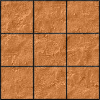#### You may also like### Pyramids

What are the missing numbers in the pyramids?### Paving the Way

A man paved a square courtyard and then decided that it was too small. He took up the tiles, bought 100 more and used them to pave another square courtyard. How many tiles did he use altogether?### Chess

What would be the smallest number of moves needed to move a Knight from a chess set from one corner to the opposite corner of a 99 by 99 square board?

# The Simple Life

##### Age 11 to 14 Challenge Level:

The suggestions in these notes are adapted from Colin Foster's article, The Simple Life

### Why do this problem?

Expanding brackets and collecting like terms to simplify expressions are perhaps not the most exciting of mathematical topics, but fluency in these skills is needed in order to solve equations and engage with more stimulating mathematical problem solving.

One way to avoid the tedium of lots of repetitive practice is to embed practice in a bigger problem which students are trying to solve. This idea is explored in Colin Foster's article, Mathematical Etudes, and this problem is an example of a mathematical etude.

### Possible approach

"I would like you to expand and simplify these four expressions. Which one is the odd one out?"
1.  $(3x + 4y) + 2(x + 2y)$
2.  $4(2x + 5y) - 3(x + 4y)$
3.  $3(2x + 3y) - (x - y)$
4.  $3(x + 3y) + (2x - y)$

When students multiply out the brackets and collect like terms they should find that (1), (2) and (4) all come to $5x + 8y$, so (3) is the odd one out. Students might get $5x + 8y$ instead of $5x+10y$ for (c) if they subtract $-y$ incorrectly.

"Did you make any mistakes? Or nearly make any mistakes?"
"What things do you have to be careful of when simplifying expressions like this?"
"Do you have any advice for someone doing questions like this?"

"Today, your challenge is to combine pairs of expressions to get $5x + 8y.$"
"The only expressions that you are allowed to use are: $$(x + y) \quad (x + 2y) \quad (x - 2y) \quad (x + 4y) \quad (2x + 3y)$$ You can pick any two of these expressions and add or subtract multiples of each.

"For example, we could choose the brackets $(x + 2y)$ and $(x + 4y)$" $$\square(x + 2y) ± \square(x + 4y) = 5x+8y$$ "Now we need to find numbers to go in the boxes."

Ask students to suggest some numbers and then work through the expanding and simplifying process together on the board. It is very unlikely that the answer will come to 5x + 8y.

"Can you choose a different pair of numbers so that we get $5x + 8y$?"
Invite the class to work on the problem in pairs. Once they find the answer, they can choose different pairs of expressions and repeat the task.

Seven pairs of expressions use whole numbers, and three pairs require fractions - see Getting Started for a list.

While they are working, circulate and look out for students using efficient strategies, like the two listed in the problem. After a while, bring the class together and invite students to talk about their methods. If no-one has approached the problem using Charlie's or Alison's methods, share them with the class.

Then give everyone a little longer to work on the problem, perhaps encouraging them to try out both methods. At the end of the lesson, you could invite students to discuss the pros and cons of each method.

You could conclude the lesson with a plenary in which the students talk about their answers and how they got them.

### Possible extension

Students could experiment with combinations of three, four or five expressions to make the target $5x+8y$.

### Possible support

Students who are not confident at manipulating algebraic expressions could try the problem Perimeter Expressions.